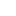v2.25ITransform3D Interface Reference

Represents a transform in the three-dimensional space, wich is a 4x4 transformation matrix. More...

Inheritance diagram for ITransform3D:## Public Member Functions

HRESULT GetElement ([in] int rowIndex, [in] int columnIndex, [out, retval]double *pValue)
Returns the component of the transformation matrix.

HRESULT SetElement ([in] int rowIndex, [in] int columnIndex, [in]double value)
Sets the component of the transformation matrix.

Adds a translation by the given vector.

HRESULT AddRotation ([in] Vector3D *axis, [in] double angle)
Adds a rotation to a given angle around the given axis.

HRESULT GetCopy ([out, retval] ITransform3D **ppCopy)
Returns a copy of the transform.

HRESULT GetMultiplied ([in] ITransform3D *pTransform, [out, retval] ITransform3D **ppResult)
Returns a transform wich is a result of the multiplication of the current transform with the specified one.

HRESULT TransformPoint ([in] Point3D *pPoint, [out, retval] Point3D *pResult)
Returns a transformed 3D point.

HRESULT TransformVector ([in] Vector3D *pVector, [out, retval] Vector3D *pResult)
Returns a transformed 3D vector.

## Detailed Description

Represents a transform in the three-dimensional space, wich is a 4x4 transformation matrix.